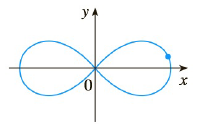Chapter 2.6, Problem 43E

Chapter
Section
Textbook Problem

# Find the points on the lemniscuses in Exercise 31 where the tangent is horizontal. 2 ( x 2 + y 2 ) 2 = 25 ( x 2 − y 2 ) , (3, 1) (lemniscuses)To determine

To find:

The points where tangent line is horizontal

Explanation

1) Concept:

Horizontal tangent has slope 0. Therefore to find the point where the function has horizontal tangent, find the derivative of curve and set it equal to zero and solve for corresponding points.

2) Formula:

ddxxn=nxn-1

3) Given :

2x2+y22=25x2-y2

4) Calculation:

2x2+y22=25x2-y2

Differentiate with respect to x

ddx2x2+y22=ddx25x2-y2

2ddxx2+y22=25ddxx2-y2

2*2x2+y2ddxx2+y2=25ddxx2-y2

4x2+y2ddxx2+y2=25ddxx2-y2

4x2+y2ddxx2+ddxy2=25ddxx2-ddxy2

4x2+y22x+2ydydx=252x-2ydydx

4*2x2+y2x+ydydx=25*2x-ydydx

4x2x+ydydx+y2x+ydydx=25x-ydydx

4x2x+ydydx+4y2x+ydydx=25x-ydydx

4x3+4x2ydydx+4xy2+4y3dydx=25x-25ydydx

4x2ydydx+4y3dydx+25ydydx=25x-4x3-4xy2

4x2y+4y3+25ydydx=25x-4x3-4xy2

### Still sussing out bartleby?

Check out a sample textbook solution.

See a sample solution

#### The Solution to Your Study Problems

Bartleby provides explanations to thousands of textbook problems written by our experts, many with advanced degrees!

Get Started

#### Solve the equations in Exercises 126. x4x2=6

Finite Mathematics and Applied Calculus (MindTap Course List)

#### Find the minimum cost C (in dollars) given that 2(1.5C + 80) 2(2.5C 20)

Applied Calculus for the Managerial, Life, and Social Sciences: A Brief Approach

#### Graph 42i. a. b. c. d.

Trigonometry (MindTap Course List)

#### True or False: is a geometric series.

Study Guide for Stewart's Single Variable Calculus: Early Transcendentals, 8th